Question

pls help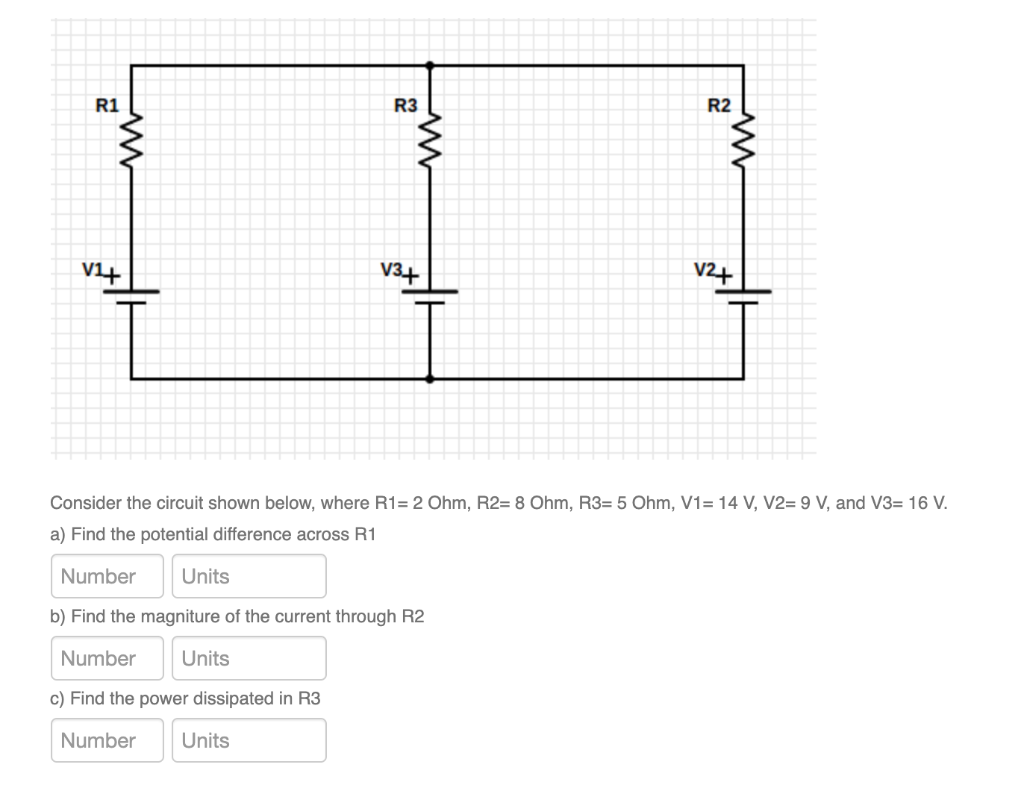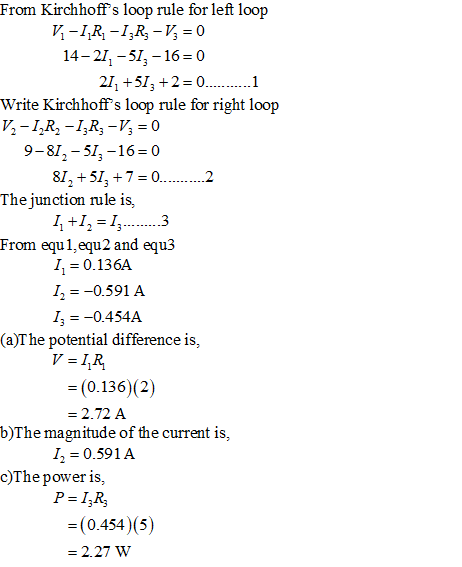#### Earn Coins

Coins can be redeemed for fabulous gifts.

Similar Homework Help Questions
• ### Consider the circuit shown below: R1 = 600 ohm R2 = 480 ohm R3 = 240...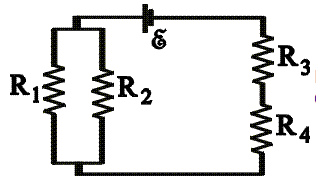Consider the circuit shown below: R1 = 600 ohm R2 = 480 ohm R3 = 240 ohm R4 = 210 ohm ε = 4.5 V a)Calculate the potential difference across R4. b)Calculate the power dissipated in R3. AA CN.

• ### For the circuit shown in the figure, If R1=512, R2=10N, R3=3N, V1= V1}V, V2=5V and V3=7V....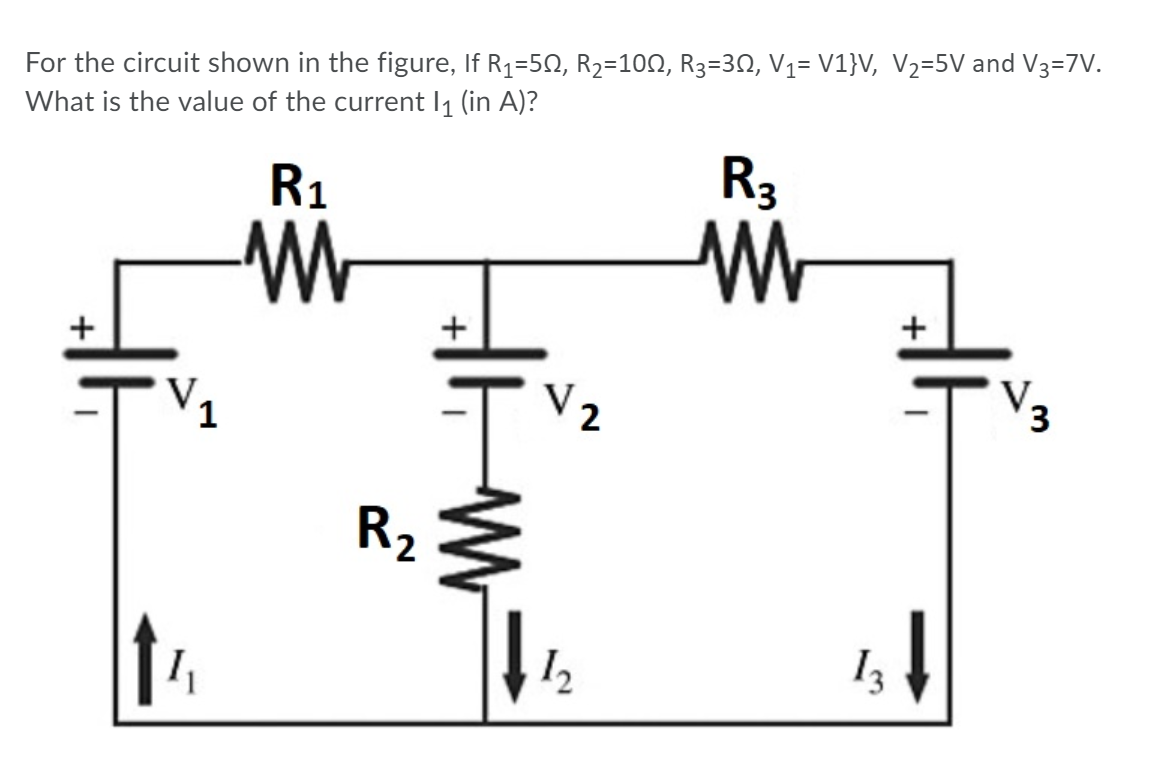For the circuit shown in the figure, If R1=512, R2=10N, R3=3N, V1= V1}V, V2=5V and V3=7V. What is the value of the current 11 (in A)? R1 -W R3 M + + V2 V. 3 R2 \$ 12 13

• ### In the circuit shown, R1 = 7 ohm, R2 = 11 ohm, R3 = 9ohm, V1...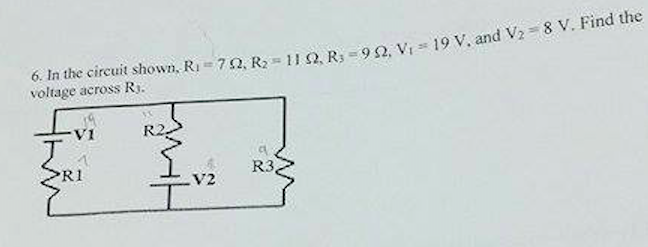In the circuit shown, R1 = 7 ohm, R2 = 11 ohm, R3 = 9ohm, V1 = 19 V, and V2 = 8V. Find the voltage across R3.

• ### R1 13 |12 Vg V2 R3 R2 18 Ohm and R3 16 Ohm. 90 V, R2...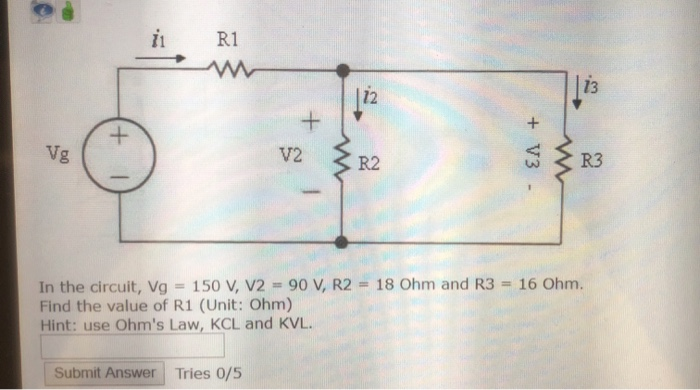R1 13 |12 Vg V2 R3 R2 18 Ohm and R3 16 Ohm. 90 V, R2 In the circuit, Vg Find the value of R1 (Unit: Ohm) Hint: use Ohm's Law, KCL and KVL 150 V, V2 Submit Answer Tries 0/5 +V3 +

• ### R1= 24.8 ohms R2= 13.9 ohms R3= 25.5 ohms V1= 5 volts V2= 11.9 volts V3=...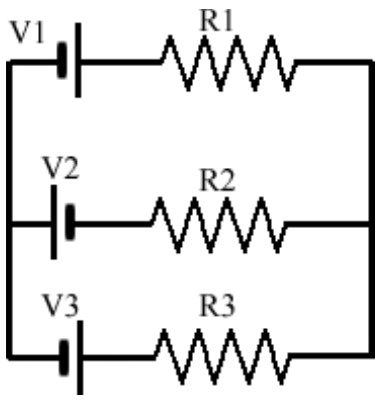R1= 24.8 ohms R2= 13.9 ohms R3= 25.5 ohms V1= 5 volts V2= 11.9 volts V3= 9.1 volts What is the current through the 13.9 ohm resistor? R1 V1 V2 R2 V3 R3

• ### In the circuit shown in the figure, VA = VB = 10.0 and R1 = R2...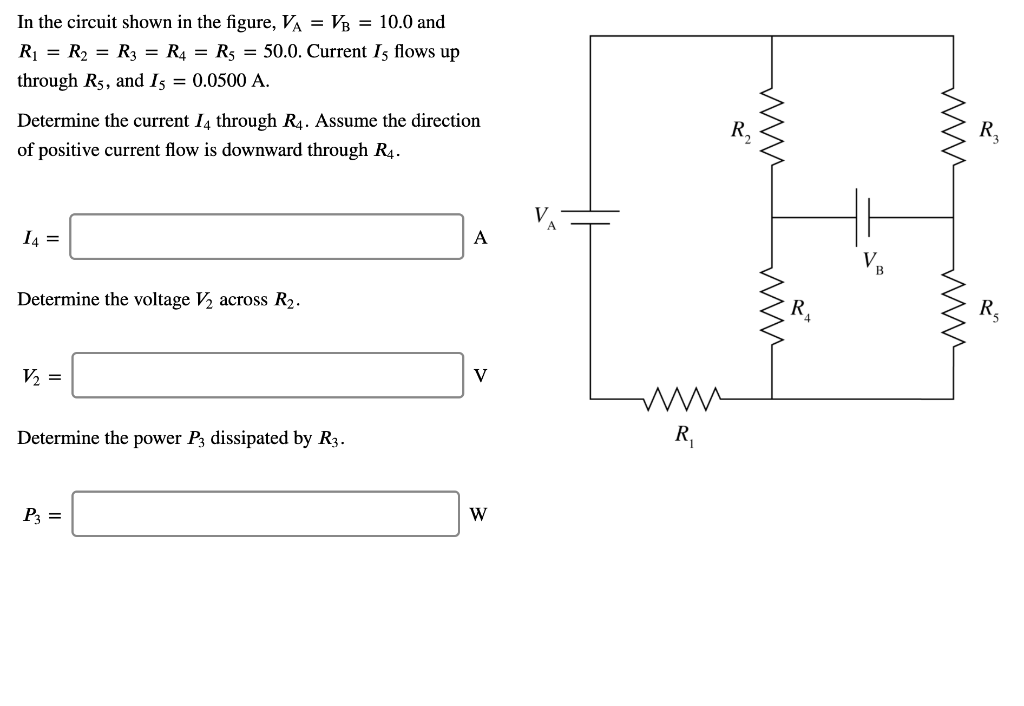In the circuit shown in the figure, VA = VB = 10.0 and R1 = R2 = R3 = R4 = Rs = 50.0. Current Is flows up through Rs, and I5 = 0.0500 A. Determine the current 14 through R4. Assume the direction of positive current flow is downward through R4. mm VA 14 = A Determine the voltage V2 across R2. mm V2 = V w Determine the power P3 dissipated by R3. R P3 = W

• ### The circuit shown in the figure below contains three resistors (R1, R2, and R3) and three...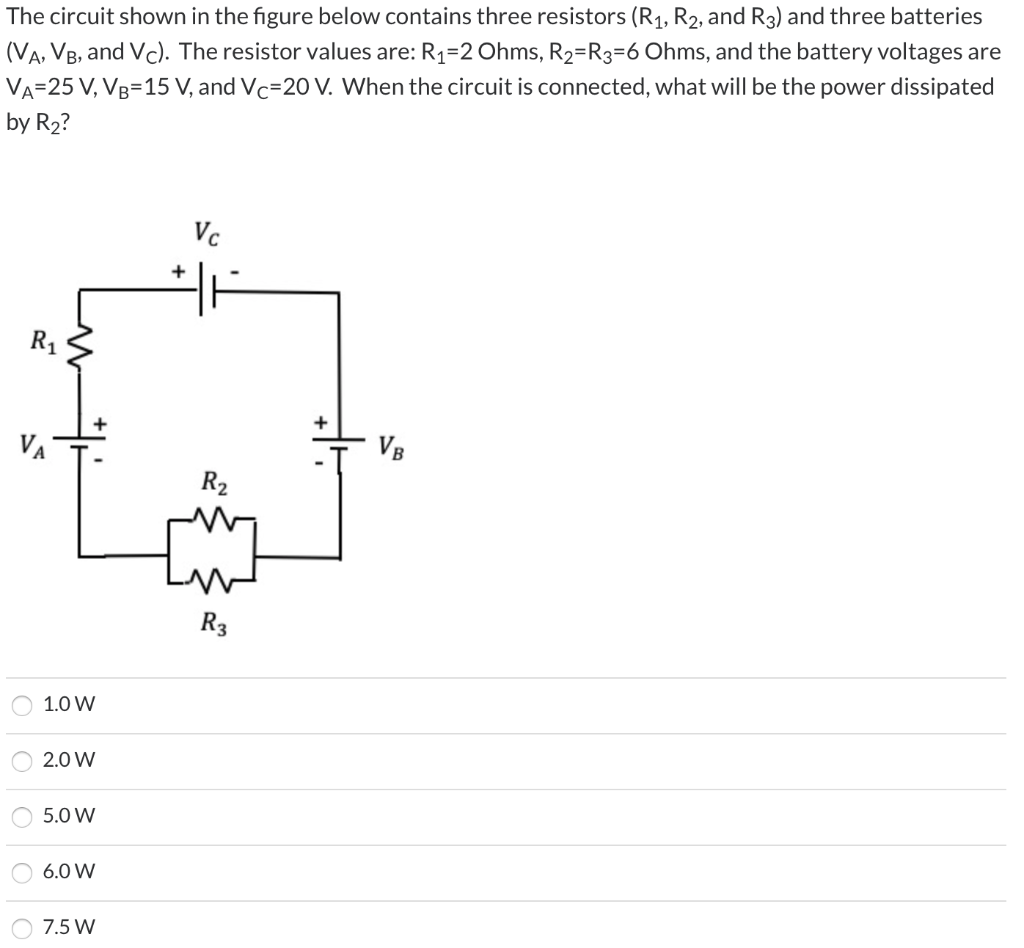The circuit shown in the figure below contains three resistors (R1, R2, and R3) and three batteries (VA, VB, and Vc). The resistor values are: R1=2 Ohms, R2=R3=6 Ohms, and the battery voltages are VA=25 V, V3=15 V, and Vc=20 V. When the circuit is connected, what will be the power dissipated by R2? Vc R1 + VA VB R2 R3 1.0 w 2.0 W 5.0 W 6.0 W 7.5 W

• ### For the following circuit, assume that R1 = 1 ohm, R2 = 2 ohms, R3 =...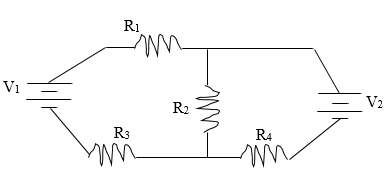For the following circuit, assume that R1 = 1 ohm, R2 = 2 ohms, R3 = 8 ohms, and R4 = 4 ohms. Also assume that V1 = 50 volts and V2 = 100 volts. Find the current through each of the resistors and the power dissipated through all of the resistors. (Hint: You’ll need to use Kirchoff’s Loop and Junction Rule) Vi R2 R3 R4 NV

• ### Consider the circuit shown in the figure with resistors R1= 4.0 Ω, R2= 3.0 Ω, R3=...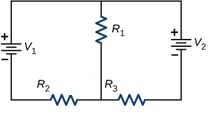Consider the circuit shown in the figure with resistors R1= 4.0 Ω, R2= 3.0 Ω, R3= 3.0 Ω, and batteries V1= 2.0 V, V2=1.5 V respectively. What is the potential drop (voltage) across resistor R3?

• ### current controlled voltage source 2i R2 V3 EV1 V2 R1 In the circuit above V1 =...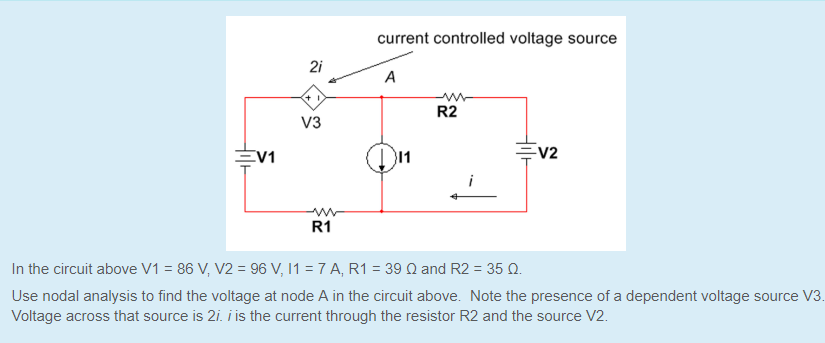current controlled voltage source 2i R2 V3 EV1 V2 R1 In the circuit above V1 = 86V,V2=96 V, 11-7AR1:39 ? and R2 : 35 ?. Use nodal analysis to find the voltage at node A in the circuit above. Note the presence of a dependent voltage source V3 Voltage across that source is 2i. i is the current through the resistor R2 and the source V2